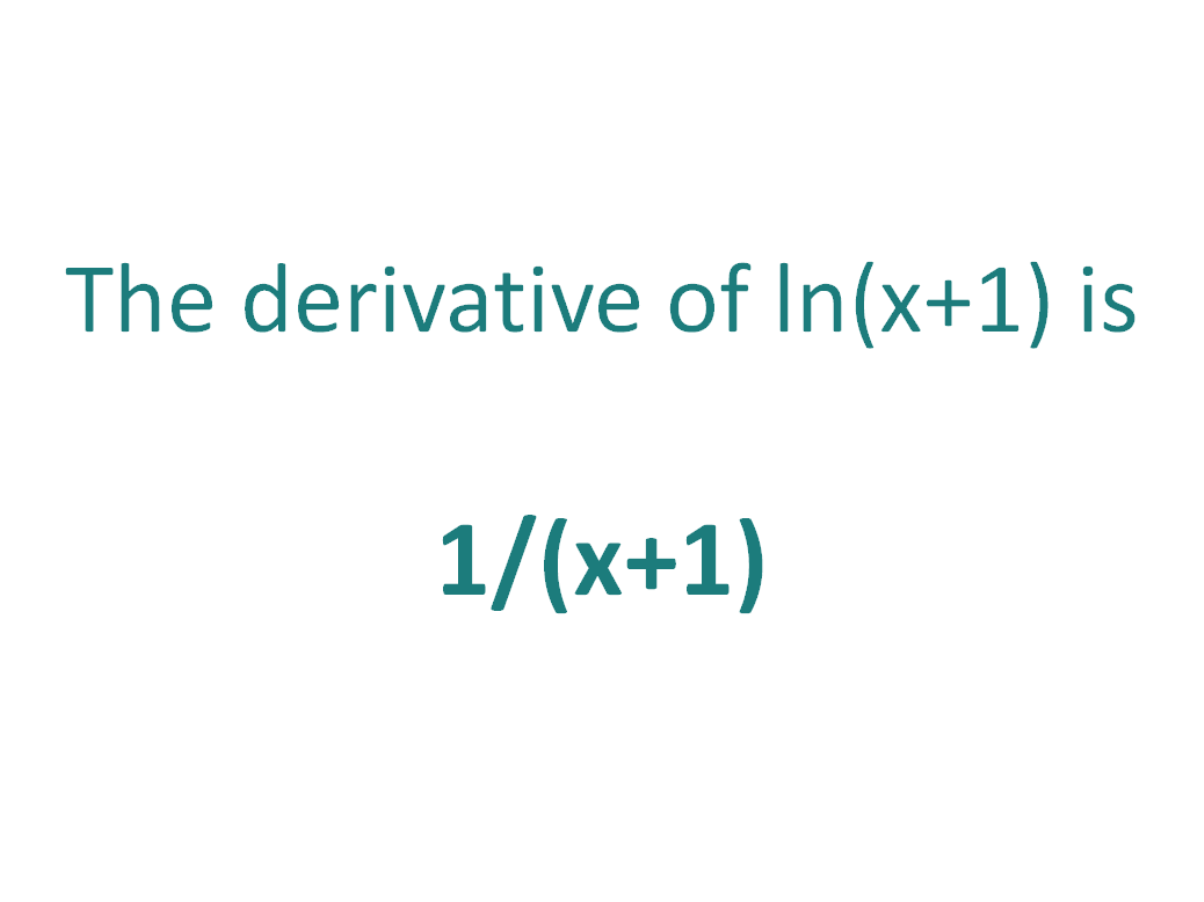# The Derivative of ln(x+1)The derivative of ln(x+1) is 1/(x+1)

## How to calculate the derivative of ln(x+1)

The chain rule is useful for finding the derivative of an expression which could have been differentiated had it been in terms of x, but it is in the form of another expression which could also be differentiated if it stood on its own.

In this case:

• We know how to differentiate x+1 (the answer is 1)
• We know how to differentiate ln(x) (the answer is 1/x)

This means the chain rule will allow us to perform the differentiation of the function ln(x+1).

To perform the differentiation, the chain rule says we must differentiate the expression as if it were just in terms of x as long as we then multiply that result by the derivative of what the expression was actually in terms of (in this case the derivative of x+1).

#### Using the chain rule to find the derivative of ln(x+1)

ln(x+1) is in the form of the standard natural log function ln(x), except it does not have x as an argument, instead it has another function of x (x+1).

Let’s call the function in the argument g(x), which means:

g(x) = x+1

From this it follows that:

ln(x+1) = ln(g(x))

So if the function f(x) = ln(x) and the function g(x) = x+1, then the function ln(x+1) can be written as a composite function.

f(x) = ln(x)

f(g(x)) = ln(g(x)) (but g(x) = x+1)

f(g(x)) = ln(x+1)

Let’s define this composite function as F(x):

F(x) = f(g(x)) = ln(x+1)

We can find the derivative of ln(x+1) (F'(x)) by making use of the chain rule.

The Chain Rule:
For two differentiable functions f(x) and g(x)

If F(x) = f(g(x))

Then the derivative of F(x) is F'(x) = f’(g(x)).g’(x)

Now we can just plug f(x) and g(x) into the chain rule. But before we do that, just a recap on the derivative of the natural logarithm.

The derivative of ln(x) with respect to x is (1/x)
The derivative of ln(s) with respect to s is (1/s)

In a similar way, the derivative of ln(x+1) with respect to x+1 is 1/(x+1).
We will use this fact as part of the chain rule to find the derivative of ln(x+1) with respect to x.

How to find the derivative of ln(x+1) using the Chain Rule:

Using the chain rule, we find that the derivative of ln(x+1) is 1/(x+1)

Finally, just a note on syntax and notation: ln(x+1) is sometimes written in the forms below (with the derivative as per the calculations above). Just be aware that not all of the forms below are mathematically correct.

## The Second Derivative of ln(x+1)

To calculate the second derivative of a function, you just differentiate the first derivative.

From above, we found that the first derivative of ln(x+1) = 1/(x+1). So to find the second derivative of ln(x+1), we just need to differentiate 1/(x+1).

We can use the quotient rule to find the derivative of 1/(x+1), and we get an answer of -1/(x+1)2

The second derivative of ln(x+1) = -1/(x+1)2